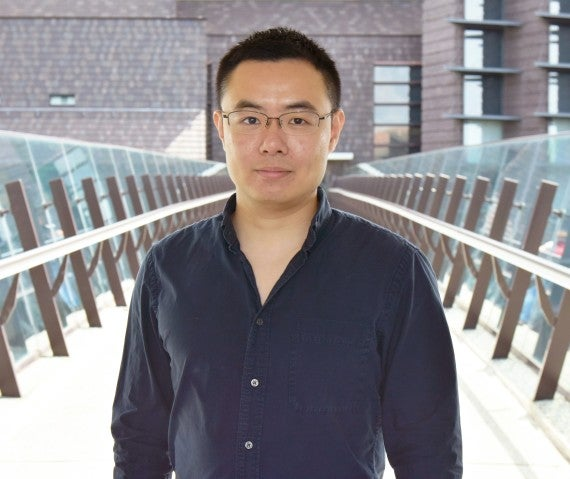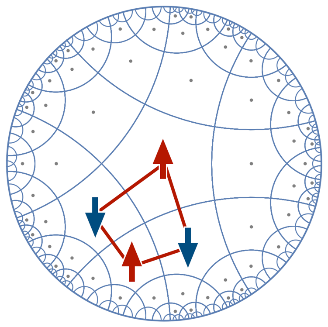# Theories Past and Present Show Quantum Connections

Study unveils connection of classical toy model to new topics in quantum gravity.

Relationships are complicated. There are boundaries, the unknown, and a lot of physics. Physics may not be the driving force for the relationships most think of, but they are in this case.

A new study by PhD student Han Yan in the Theory of Quantum Matter Unit at the Okinawa Institute of Science and Technology Graduate University (OIST) connects theories, past and present, unveiling the relationship of gravity to a classic toy model of fracton state of matter. The work was published on April 15, 2019 as an Editors’ Suggestion in Physical Review B.PhD Student Han Yan in the Theory of Quantum Matter Unit at the Okinawa Institute of Science and Technology Graduate University (OIST) recently published a paper in Physical Review B.

The toy model used by Yan is in a space of negative curvature, like an hourglass shape. In recent years, scientists have become more interested in this type of model because they harbor new exotic states of matter, dubbed as ‘fracton states.’ In the study, Yan found that fracton models satisfy holographic properties – implying that this model mimics how gravity transfers information.

Holography is a duality between physics in higher dimension and lower dimension. In the higher dimensional space, gravity exists so there can be black holes and other exotic gravitational effects. In the lower dimensional space, there is no gravity – only conventional quantum mechanics.

“Holography is a very surprising property of gravity,” Yan said. “That is, quantum gravity is equivalent to another system living on its boundary without gravity. Quantum gravity and black holes are very difficult to understand, but with the help of holography we can study its boundary equivalence instead.”

In Yan’s work, he puts the fracton model in a curved space – where holography works for gravity. Yan checked if the properties also work for fracton models, and they did.

In the late 1600s, Sir Isaac Newton discovered gravity, leaving theorists and scientists to investigate the force that affects us all. As technology and understanding advance, the puzzle of understanding such theories proves testing.

“Holography is really important for our understanding of quantum gravity,” Yan said. “Gravity is very hard to understand because of all the quantum effects of black holes. Knowing it is equivalent to a normal quantum mechanics system opens a lot of gateways.”

The toy model used by Yan is quite simple, but it displays the most essential holographic properties. However, simplification does not mean easy. When putting the model in a curvature of space time, the physics can be easily lost. Before Yan puts the model in a curved space, it is a flat lattice of squares. Once curvature is introduced, the squares turn to pentagons, as the pentagons spin, sections of the lattice change color.

PhD Student Han Yan in the Theory of Quantum Matter Unit at the Okinawa Institute of Science and Technology Graduate University (OIST) put a fracton model in a curved space – where holography works for gravity. Yan checked if the properties also work for fracton models, and they did.

Think of synchronized swimmers scattered in a pentagon-like pattern, all spinning in the ocean of curved space. They coordinate with their neighbors in a way so that a swimmer at one end can influence another one at the other end. The synchronization of the swimmers transfers information in a manner like gravity.A hyperbolic lattice used by Han Yan in his most recent study. The lattice display pentagons changing their states collectively, which mimic gravitational forces.

“This is the first many body model that mimics gravity when you put it in a curved spacetime and there were none before,” Yan said. “To me, the fact that the fracton model mimics gravity is the most significant part. It is a cool side product that we may simulate gravity in the lab – but there is still a long way to go.”

Specialties

Research Unit